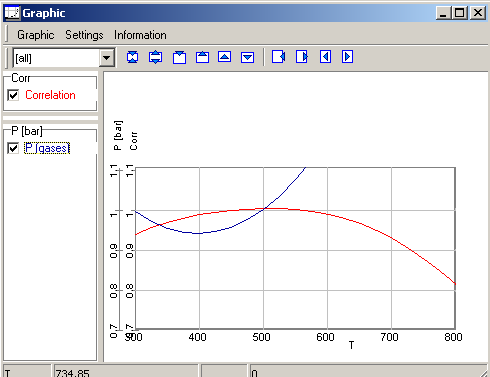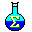5. Recalculator

Content
5.1. General
5.3. Possible Errors and Solutions
5.4. Examples

5.1. General

When several values of the concentrations are known, the application calculates the other concentrations in an equilibrium system corresponding known concentrations. The possible temperature and/or pressure can be estimated in an equilibrium state corresponding to given conditions or  measurements.

First, the elements and compounds of the system are to select. When reference concentrations are written in the P(m) and/or a (m) columns and checked as reference, a calculation can be carried out. The result of the calculation are written by the application in the f(c) and a(c) columns.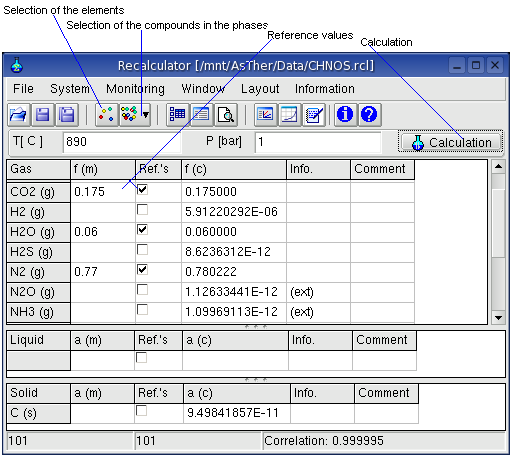Preconditions for a calculation
The number of the reference concentrations should be as much as  the number of the elements in the system. It is recommended, that the number of the reference concentrations should me more then the number of the elements. If the number of the references values is equal to the number of the elements, than the correlation coefficient will be 1,  and has no statistical significance. All elements must be contained at least in one reference values.

Although the thermodynamic system is displayed in the user interface in three phases, the real system can consist of more than three phases. You can, e.g. calculate the activities of solids in separate solid phases.

All the elements or substances have not to be regarded in a calculation. For example,  if a systems contains the elements C, H, N, O, Zn, Pb (or more), a calculation can be carried out regarding C, H, O, when the compounds are used as reference, which consist of C, H, O. In a such case, it is not possible to check the total system pressure. Partial pressures or activities, which are zero, can not be regarded as reference.

Value of the correlation coefficient (0 to 1) means
0:  the result is inconsistent according to the inputs
1:  best possible result
For a reliable calculation the following should apply: - the correlation coefficient should be > 0.9 - the total of the calculated individual partial pressures of the system should be equal to the measured total pressure if all components of the gas phase are considered in the calculation.

System -> Elements: the elements to be considered in the calculation can be selected from a dialog box.System -> Compounds -> Gas,   System -> Compounds -> Liquid  and System -> Compounds -> Solid: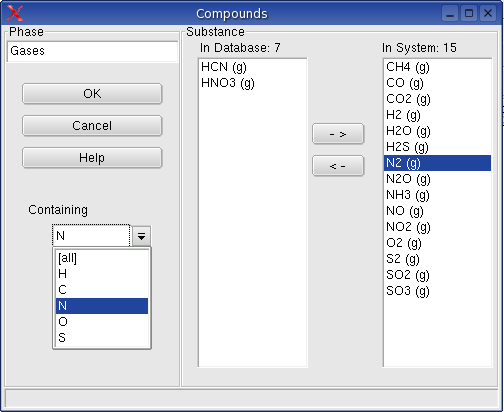When the elements have been selected, the possible components available in the database are listed in a dialog box for consideration in the system.

The drop-down-box Containing enables to constrict the shown substances in the Database-Box
In the picture above, when N is selected in the drop down box, only substances will be shown in the Database-Box, which contains N, and which is consisting from C, H, O, N, S: corresponding to the element selection.

System -> Behaviour: You can select, according to what law the gases should be treated. The state functions can be calculated according to the law of real gases if the critical data ( Tc, Pc ) of the gases are known.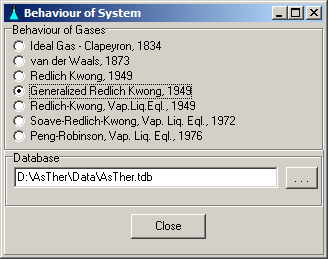System-> Calculation: carries out a calculation.
System-> Calculation by Variation: Several calculations will be subsequently carried out according to a given variation of parameters. Such calculations are especially useful for the tabular and graphical display of the calculation resultsMonitoring -> Record Variables: A dialog box will be shown, in which the record variables can be selected.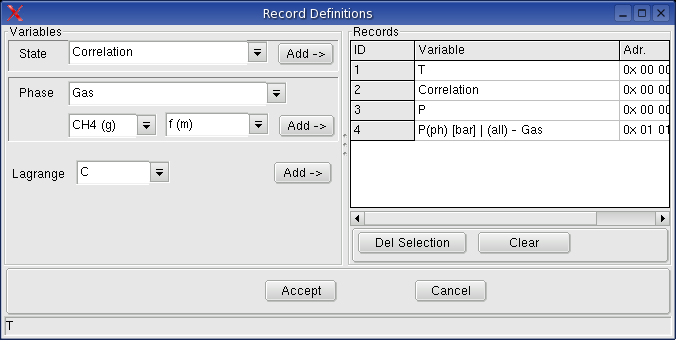When a variable is selected to record, the entered and/or calculated values of the variables written in an table, when a calculation is executed.

Monitoring -> Record Table the table will be shown, in which the calculated values of the selected record variables are written by application.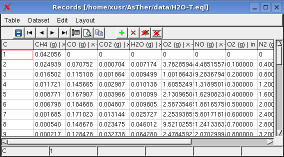Monitoring  -> Graphic Variables

When calculation variables are recorded, the data of the record table can be shown in a graphic window.
You can define, which recorded variables are to show in the graphic window.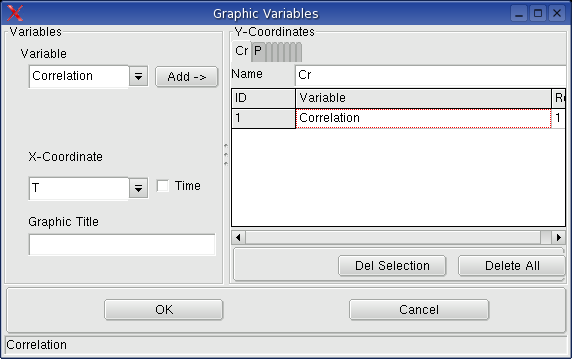Monitoring -> Graphic: shows the graphic window.
More About Graphic Windows in Cap.7.

5.3. Errors and Solutions
Linear Dependence: The errors are caused, when the number of the reference concentrations are less then the number of the elements in the system.
A system consisting of H2O(g), H2(g), O2(g) and H2O(l), needs at last two references because two elements H and O.
Only the reference values for H2O (l) and H2O(g) causes the error linear dependence.  The reference values for O2(g) and H2O(l) are sufficient for a calculation.

5.4. Examples
Calculation of the composition of a gas phase and its temperature

A system consists of the elements C, O, H, N.
Following measurements are knownSoot (carbon) formation is observed.
The average temperature is expected between 300 �C and 800 �C
Based on these measurements, the partial pressures for CO- and NOx- as well as the temperature of the system 1 bar will be calculated as follows:

5.4.1. Selection of the elements of the system: menu System -> Elements:
select H, C, N, O

5.4.2. Selection of components in the phases: menu  System -> Compounds -> Gases
Enter the given values and activate as Reference as following picture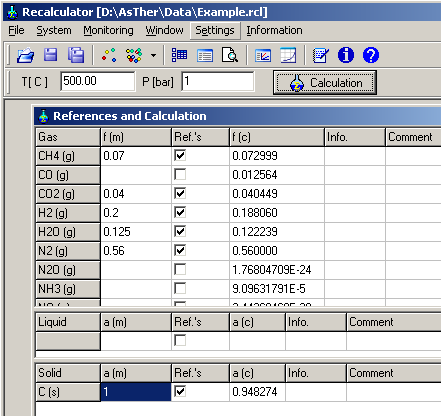5.4.3. Definition of Record Variables: menu Monitoring -> Record variables
Select the variable Temperature and Correlation as record variable5.4.3. Calculations: Menu System -> Calculation by Variation

Execute calculation from 300�C by steps 25�C to 800�C.
The results of the calculations.The calculations yield max. correlation as 500�C. The corresponding pressure of system is 0.9964 bar.
The phase pressure can be real system pressure, when all compounds of real system regarded in the calculation. The following picture shows the graphic of the results